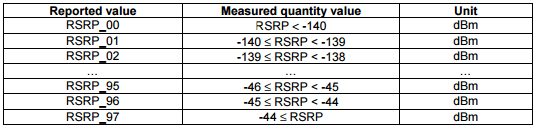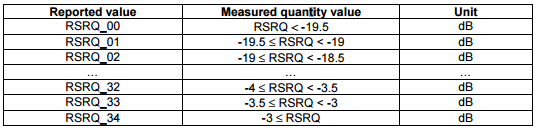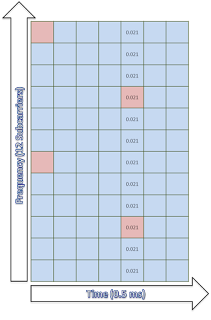## Pages

In LTE network, UEs need to measure signal strength of its own and neighbor cells constantly, during idle, connected mode or handovers in order to keep the signal quality constant. UE measures RSRP and RSRQ in LTE

#### Reference Symbol Received Power (RSRP):

• RSRP is the linear average of the downlink reference signals across the channel bandwidth
• RSRP provides information about signal strength and  gives no indication of signal quality
• RSRP measurements are used in handover, cell selection and cell re-selections
• The reporting range of RSRP is defined from -140 dBm to -44 dBm with 1 dB resolution as shown in table belowRSRP measurement report mapping (3GPP Reference: TS 36.133)

• RSSI is measured only in symbols containing Reference signals
• RSSI includes power from serving cell as well as co-channel interference and noise
• RSSI helps in determining interference and noise information
• RSSI is never reported by UE

#### Reference Signal Received Quality (RSRQ):

• RSRQ indicates quality of received reference signal. RSRQ measurement and calculation is based on RSRP and RSSI since RSRP determines signal quality and RSSI determines co-channel interference and noise. RSRQ formula is shown below (N represents number of resource blocks)
• The reporting range of RSRQ is defined from -19.5 dB to -3 with 0.5 dB resolutionRSRQ measurement report mapping (3GPP Reference: TS 36.133)

#### ExampleLets try to calculate RSRP, RSSI and RSRQ for one very simple case of one resource block with 12 sub carriers and 0.5 ms in time domain. For sake of simplicity, lets assume the power of reference symbols  (shown by red square) and power of other symbols carrying other data channels (shown by blue square) is same i.e. 0.021 watt

Since RSRP is linear average of downlink reference signal for given channel bandwidth therefore
RSRP = 10*log (0.021*1000) = 13.2 dBm

While RSSI is total received wide-band power. Therefore we have to add power of all 12 carriers in the given resource block
RSSI = 10*log(0.021*1000)+10*log(12) = 24 dBm

RSRQ is now simple ratio of RSRP to RSSI with N=1
RSRQ = 10*log(0.021/(12*0.021)) = -10.79 dB

#### Why do we use dBm as a unit of Power

We use decibels-milliwatts to measures power levels in telecommunication and other fields instead of Watt. The reason to use logarithmic scale is that it helps in reducing massive values to smaller number

Example : 0.00000000000080 watt which apparently looks very small value but
can still be received by antenna. The logarithmic value is just -91 dbm by using below formula
P (dBm) = 10 x Log (1000*P)

#### dBm vs dB

dB is ratio between two power values while dBm is used to express an absolute value of power. So when we mention RSRP and RSSI we shall always use dBm since we are talking about absolute power values but we need to use dB with RSRQ since it is the ratio of RSRP to RSSI